# GMAT Math : DSQ: Calculating median

## Example Questions

← Previous 1

### Example Question #56 : Descriptive Statistics

What is the median number of students assigned per workshop at School R?

(1) 30% of the workshops at School R have 6 or more students assigned to each workshop.

(2) 40% of the workshops at School R have 4 or fewer students assigned to each workshop.

B. Statement (2) ALONE is sufficient, but statement (1) alone is not sufficient.

D. EACH statement ALONE is sufficient.

C. BOTH statements TOGETHER are sufficient, but NEITHER statement ALONE is sufficient.

A. Statement (1) ALONE is sufficient, but statement (2) alone is not sufficient.

E. Statements (1) and (2) TOGETHER are NOT sufficient.

C. BOTH statements TOGETHER are sufficient, but NEITHER statement ALONE is sufficient.

Explanation:

Looking at statements (1) and (2) separately, we cannot get the median number since we don’t know the 50th percentile. However, putting the two statements together, we know that the 50th percentile is 5. So the median is 5.

### Example Question #1 : Median

The median of the numbers,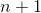,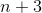, and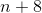is. What isequal to?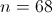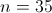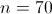None of the other answers are correct.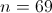Explanation:

The four numbers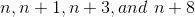appear in ascending order, so their medianmust be the arithmetic mean of the middle two numbers. Therefore,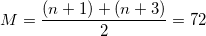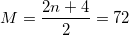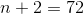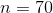### Example Question #58 : Descriptive Statistics

What is the mean of this set?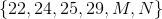1)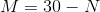2)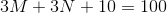BOTH statements TOGETHER are sufficient to answer the question, but NEITHER statement ALONE is sufficient to answer the question.
Statement 2 ALONE is sufficient to answer the question, but Statement 1 ALONE is not sufficient to answer the question.
BOTH statements TOGETHER are not sufficient to answer the question.
Statement 1 ALONE is sufficient to answer the question, but Statement 2 ALONE is not sufficient to answer the question.
EITHER statement ALONE is sufficient to answer the question.
Explanation:

If, then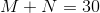.

If, then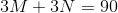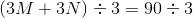The two statements are equivalent. This is enough to allow the mean to be found: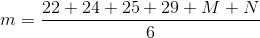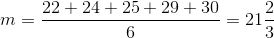The answer is that either statement alone is sufficient to answer the question.

### Example Question #2 : Median

Data Sufficiency Question

The mean of 8 numbers is 17. Is the median also 17?

1. The range of the numbers is 11.

2. The mode is 17.

statement 1 alone is sufficient, but statement 2 alone is not sufficient to answer the question

each statement alone is sufficient

both statements taken together are sufficient to answer the question, but neither statement alone is sufficient

statement 2 alone is sufficient, but statement 1 alone is not sufficient to answer the question

statements 1 and 2 together are not sufficient, and additional data is needed to answer the question

statements 1 and 2 together are not sufficient, and additional data is needed to answer the question

Explanation:

The range tells us the difference between the maximum and the minimum values, but provides no information about the median. The mode indicates the number that appears most frequently in the data set. While it is possible that the median is 17, it is impossible to determine the median from the data provided.

### Example Question #60 : Descriptive Statistics

On Monday, 40 people are asked to rate the quality of product A on a seven point scale (1=very poor, 2=poor.....6=very good, 7=excellent).

On Tuesday, a different group of 40 is asked to rate the quality of product B using the same seven point scale.

The results for product A:

7 votes for category 1 (very poor);

6 vote for 4;

The results for product B:

2 votes for category 1 (very poor);

6 vote for 4;

It appears that B is the superior product.

Which one of the following statements is true?

The mean score of product A is greater than the mean score of product B.

The median score of product A is greater than the median score of product B.

The median score for product A is less than the mean score for product A.

The median score of product A is greater than the mean score of product A.

The median score of product B is less than the mean score of product B.

The median score for product A is less than the mean score for product A.

Explanation:

Median of A = 3

Mean of A = 3.2

Median of B = 5

Mean of B = 4.8

### Example Question #61 : Descriptive Statistics

What is the median of the following numbers?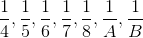Statement 1: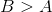Statement 2: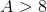and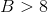Statement 2 ALONE is sufficient to answer the question, but Statement 1 ALONE is NOT sufficient to answer the question.

BOTH statements TOGETHER are sufficient to answer the question, but NEITHER statement ALONE is sufficient to answer the question.

Statement 1 ALONE is sufficient to answer the question, but Statement 2 ALONE is NOT sufficient to answer the question.

EITHER statement ALONE is sufficient to answer the question.

BOTH statements TOGETHER are insufficient to answer the question.

Statement 2 ALONE is sufficient to answer the question, but Statement 1 ALONE is NOT sufficient to answer the question.

Explanation:

Statement 1 alone would not be helpful.

Example 1: If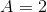and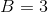, the list, in descending order, is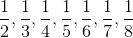and the median would be.

Example 2: If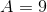and, the list, in descending order, is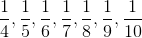and the median would be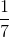.

In contrast, if Statement 2 is true, sinceand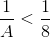and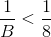. Regardless of their relationship, this makesthe fourth-highest number, and, therefore, the median.

### Example Question #62 : Descriptive Statistics

Consider the data set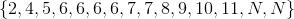What is the value of?

Statement 1: The data set is bimodal.

Statement 2: The median of the data set is 7.

BOTH statements TOGETHER are insufficient to answer the question.

Statement 1 ALONE is sufficient to answer the question, but Statement 2 ALONE is NOT sufficient to answer the question.

BOTH statements TOGETHER are sufficient to answer the question, but NEITHER statement ALONE is sufficient to answer the question.

EITHER statement ALONE is sufficient to answer the question.

Statement 2 ALONE is sufficient to answer the question, but Statement 1 ALONE is NOT sufficient to answer the question.

Statement 1 ALONE is sufficient to answer the question, but Statement 2 ALONE is NOT sufficient to answer the question.

Explanation:

Statement 1 is sufficient to prove that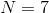. If, then each of 6 and 7 occurs four times, more than any other element, making the set bimodal. If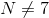, then no element other than 6 occurs more than three times, giving the set only one mode.

Statement 2 is insufficient. The median of this data set, which has fifteen elements, is its eighth-greatest element. This happens if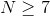.

For example, if, the set becomes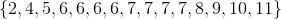If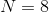, the set becomes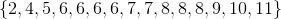The median of both sets is 7.

### Example Question #1 : Dsq: Calculating Median

A data set comprises thirteen elements, the median of which is 75. Two new elements are added to the data set. Does the median change?

Statement 1: One of the elements added to the data set is 30.

Statement 2: One of the elements added to the data set is 40.

EITHER statement ALONE is sufficient to answer the question.

BOTH statements TOGETHER are insufficient to answer the question.

Statement 2 ALONE is sufficient to answer the question, but Statement 1 ALONE is NOT sufficient to answer the question.

BOTH statements TOGETHER are sufficient to answer the question, but NEITHER statement ALONE is sufficient to answer the question.

Statement 1 ALONE is sufficient to answer the question, but Statement 2 ALONE is NOT sufficient to answer the question.

BOTH statements TOGETHER are insufficient to answer the question.

Explanation:

The median of a data set with thirteen elements is the seventh-highest element; the median of a data set with fifteen elements is the eighth-highest.

The two statements together provide insufficient information, as we show with two examples.

The data set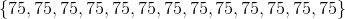has thirteen elements and median 75; after 30 and 40 are added, the set is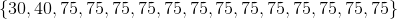,

which has median 75.

By contrast, the data set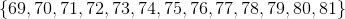has thirteen elements and median 75; after 30 and 40 are added, the set is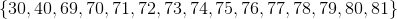which has median 74.

Both sets started out with median 75, but the addition of 30 and 40 changed one median and not the other.

### Example Question #64 : Descriptive Statistics

A data set comprises eleven elements, the median of which is 60. Two new elements are added to the data set.

Does the median change?

Statement 1: One of the elements added to the set is 50.

Statement 2: One of the elements added to the set is 70.

EITHER statement ALONE is sufficient to answer the question.

BOTH statements TOGETHER are insufficient to answer the question.

Statement 2 ALONE is sufficient to answer the question, but Statement 1 ALONE is NOT sufficient to answer the question.

Statement 1 ALONE is sufficient to answer the question, but Statement 2 ALONE is NOT sufficient to answer the question.

BOTH statements TOGETHER are sufficient to answer the question, but NEITHER statement ALONE is sufficient to answer the question.

BOTH statements TOGETHER are sufficient to answer the question, but NEITHER statement ALONE is sufficient to answer the question.

Explanation:

If we only assume Statement 1, then by examining these two cases, we show that we do not know whether the median changes.

Suppose the original set is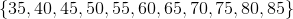If we only know that one of the elements added is 50, then it is possible that the median does not change - this happens if the other element added is 70: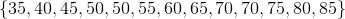has median 60.

It is also possible that the median does change - this happens if the other element added is 50.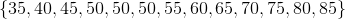has median 55.

A similar argument can be used to demonstrate that Statement 2 is insufficient.

However, suppose we know both. 60 is the sixth-highest element of the old data set, and, since one element greater than 60 and one less than 60 are added, 60 is the seventh-highest element of the new thirteen-element set. We therefore know the median did not change.

### Example Question #65 : Descriptive Statistics

Given five distinct positive integers -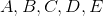- which of them is the median?

Statement 1: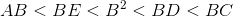Statement 2: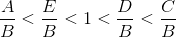BOTH STATEMENTS TOGETHER provide sufficient information to answer the question, but NEITHER STATEMENT ALONE provides sufficient information to answer the question.

BOTH STATEMENTS TOGETHER do NOT provide sufficient information to answer the question.

EITHER STATEMENT ALONE provides sufficient information to answer the question.

STATEMENT 1 ALONE provides sufficient information to answer the question, but STATEMENT 2 ALONE does NOT provide sufficient information to answer the question.

STATEMENT 2 ALONE provides sufficient information to answer the question, but STATEMENT 1 ALONE does NOT provide sufficient information to answer the question.

EITHER STATEMENT ALONE provides sufficient information to answer the question.

Explanation:

Both statements can be shown to be equivalent to the continued inequality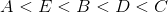by way of the Multiplication Property of Inequality. We will demonstrate this as follows:

Multiply each expression in(Statement 1)

by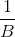: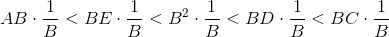.

Multiply each expression in(Statement 2)

by: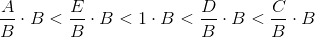The median of five numbers (an odd number) is the number in the middle when they are arranged in ascending order. Therefore, it can be established from either statement alone that of the five, the median is.

← Previous 1

Tired of practice problems?

Try live online GMAT prep today.